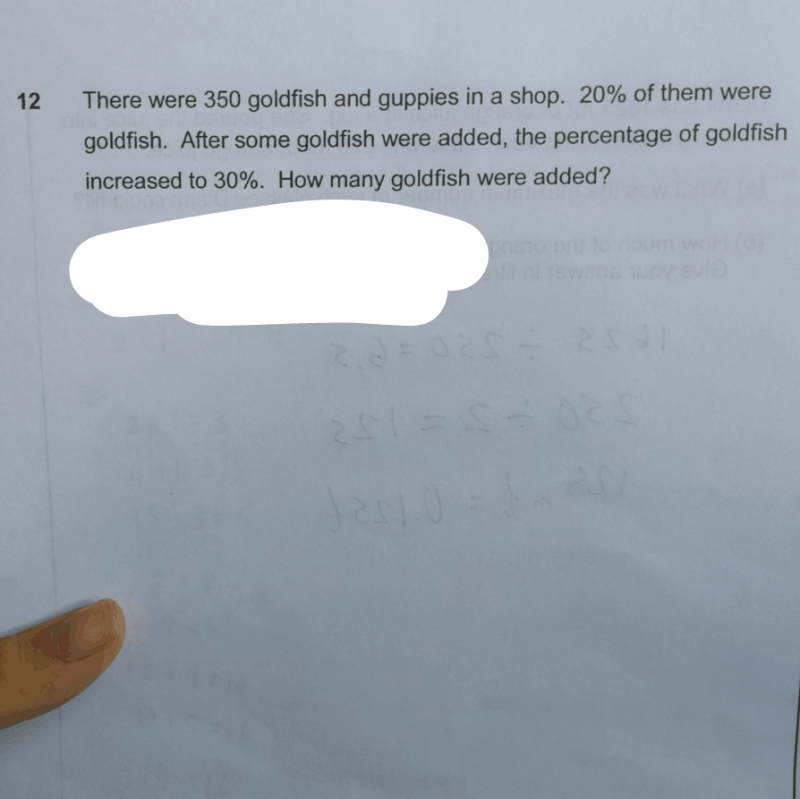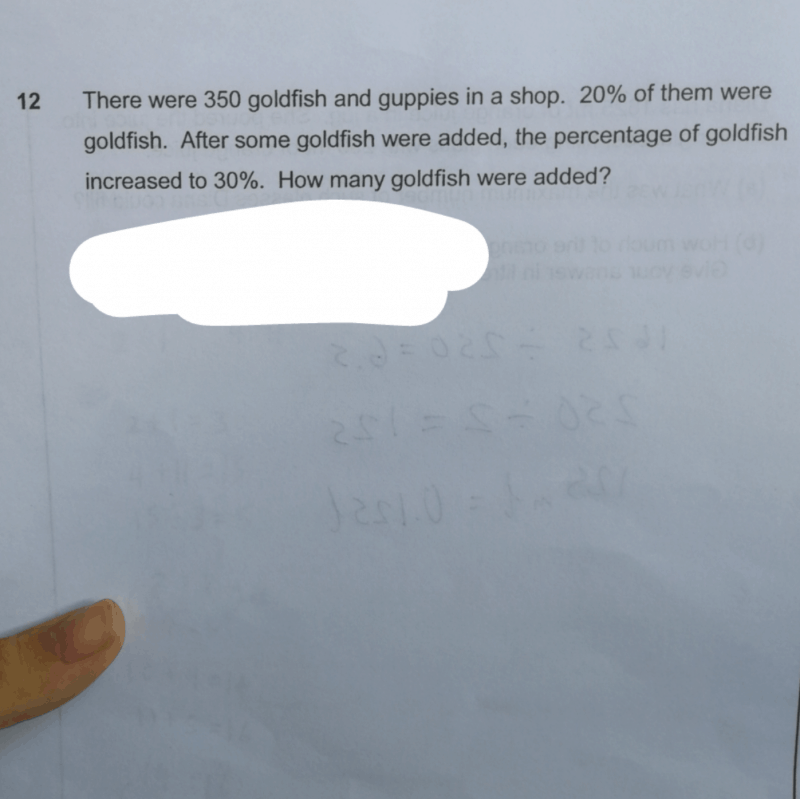# QuestionPlease help

1 Answer

# AnswerFrom the question, we know that the number of guppies is unchanged and only the number of goldfish is changed.

So, we know that 80%=280(at first)for guppies and 70%=280(later on).

Also, we know that 20%=70(at first)for goldfish and 30%=120(later on), which can be found out using the calculator.

With this,we can then find out the difference between the number of goldfish at first and later on, which is 50, the answer.

0 Replies 1 Like ✔Accepted Answer